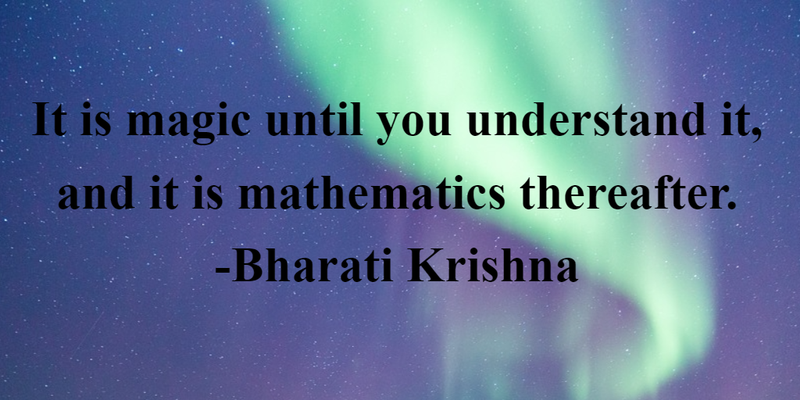Let’s face it, when you heard you’ll need math every day of your life in school from your math teacher, you would roll your eyes. While you may not realize it, you use math in a great number of ways even with the simplest of tasks. From cooking to calculating how long it will take you to get to work; you do actually use math every day. These mathematics quotes will have you question just how often you implement math equations into your daily activities.

## Quotes on Mathematics Applied to Everyday Life

1

There is math in all of God’s creations.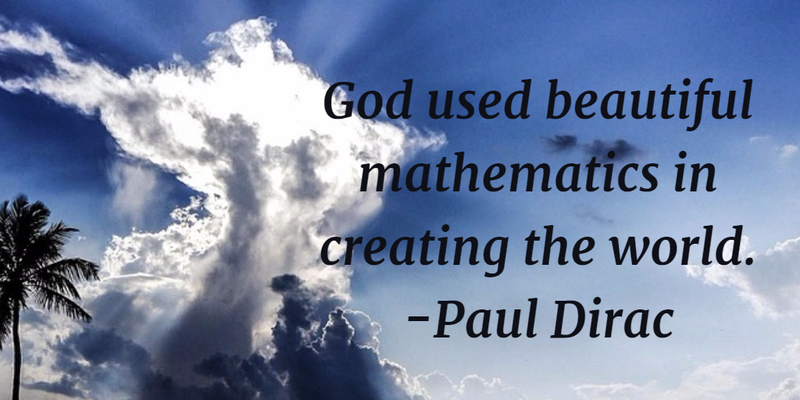2

Everything you look at can be explained with math.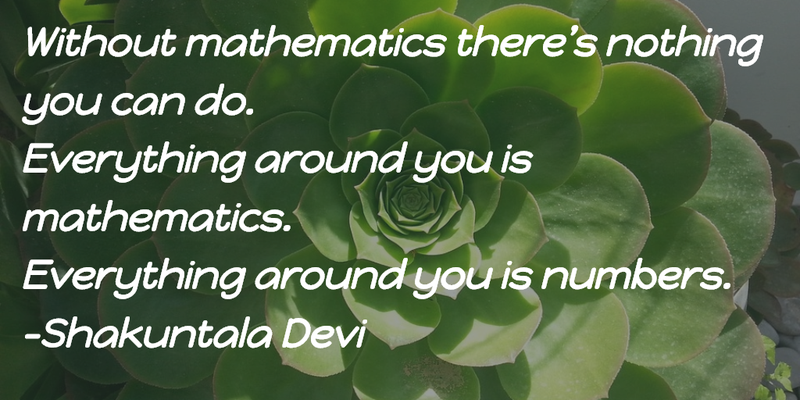3

The laws of mathematics.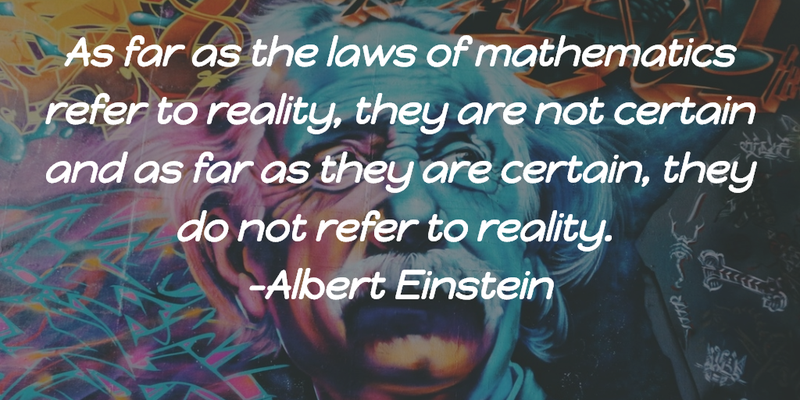4

How do you define mathematics?5

Do you agree with his words?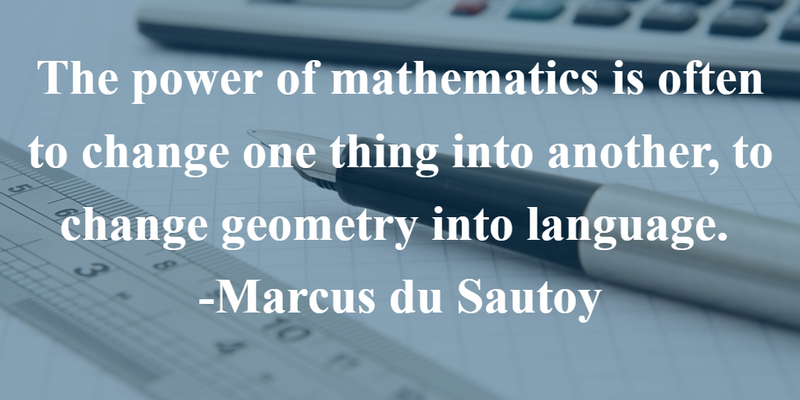6

Math is like music.7

Mathematics is not boring, well if you like it...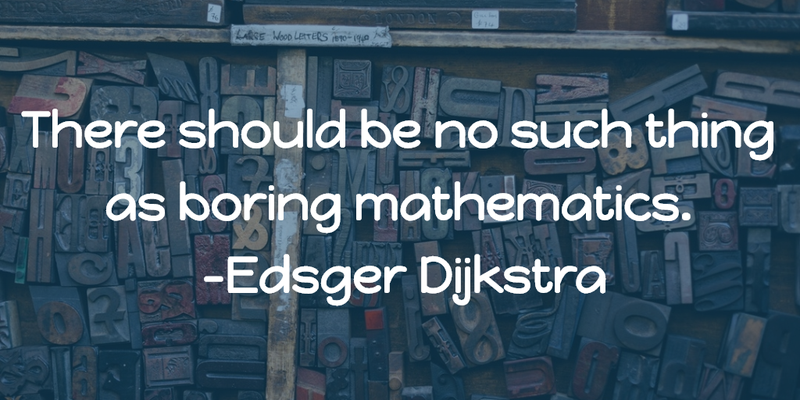89

This is a common problem.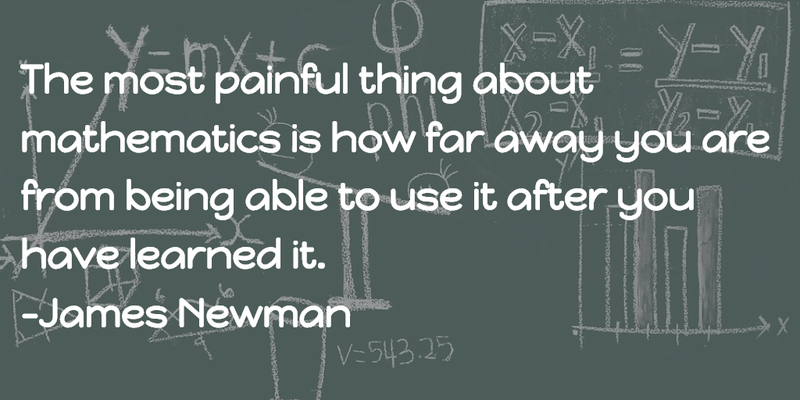10

Nowadays, technology is making math much easier.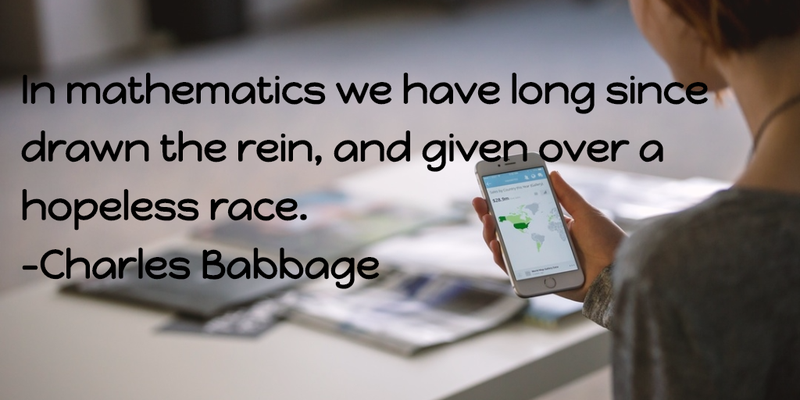11

There are a lot of rules when it comes to mathematics.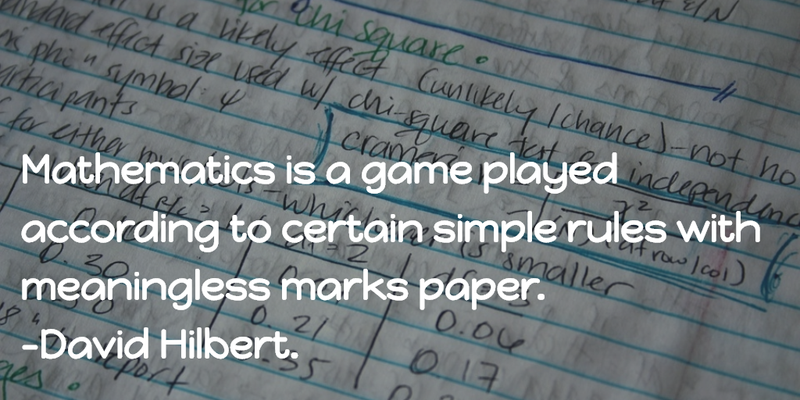12

It is obvious that most of these quotes are from mathematicians.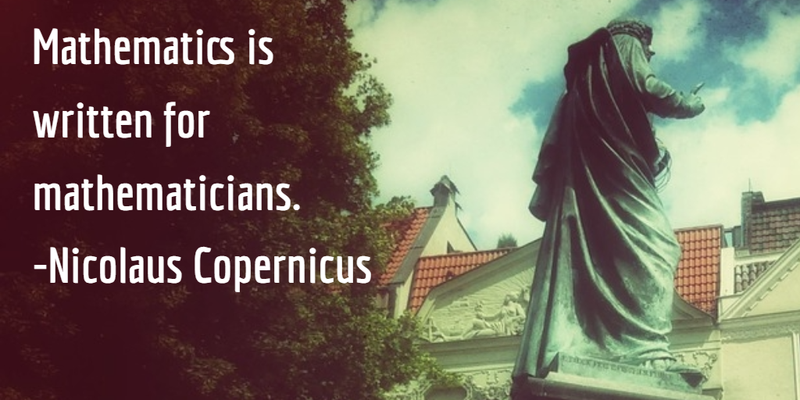13

Every word has its specific place in mathematics.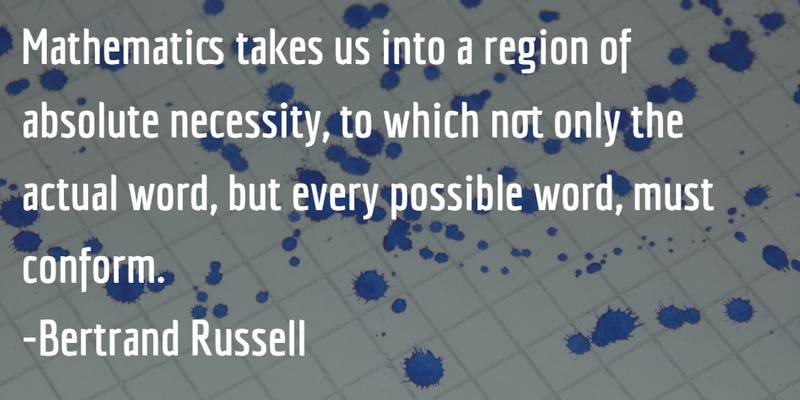14

Balance and order are key in mathematics.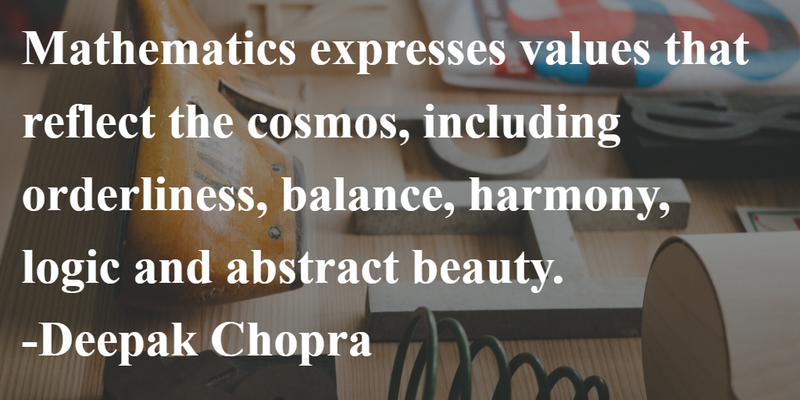15

Mathematicians vs. poets.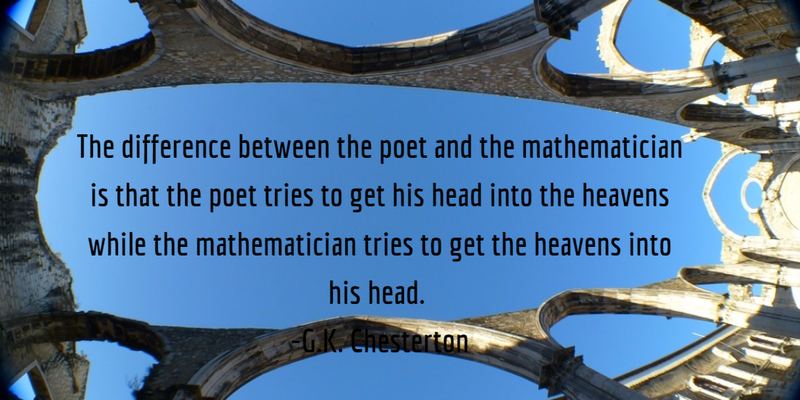16

Yes, every problem has a solution.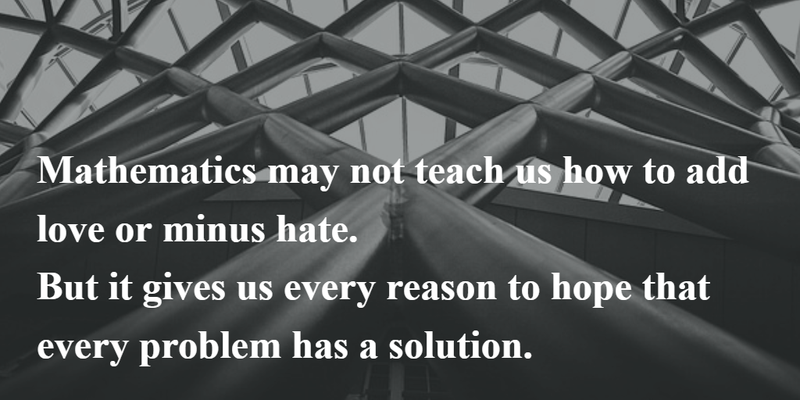17

How many math problems can you explain to someone else?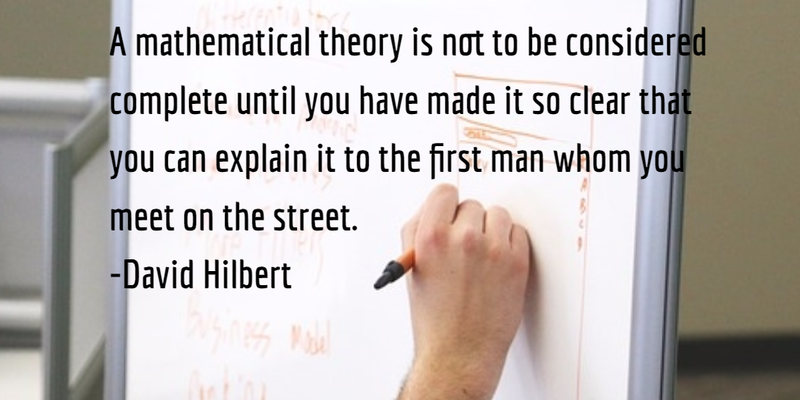18

Math is much simpler than life.19

Find fun in mathematics.20

There is often confusion with mathematics.21

This is probably why there is so much confusion.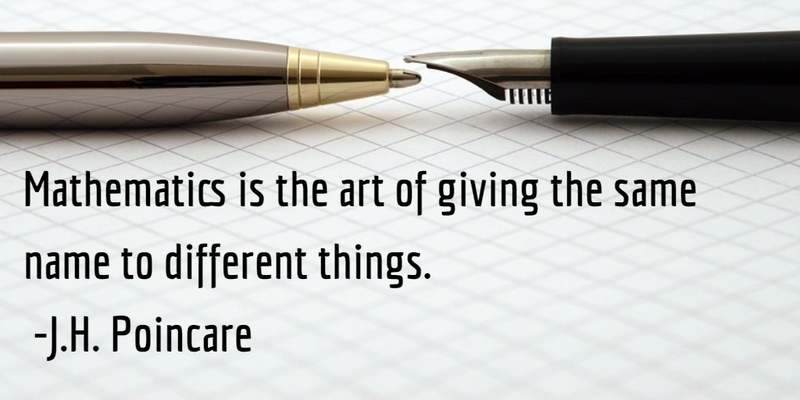22

There are always extra steps with mathematics.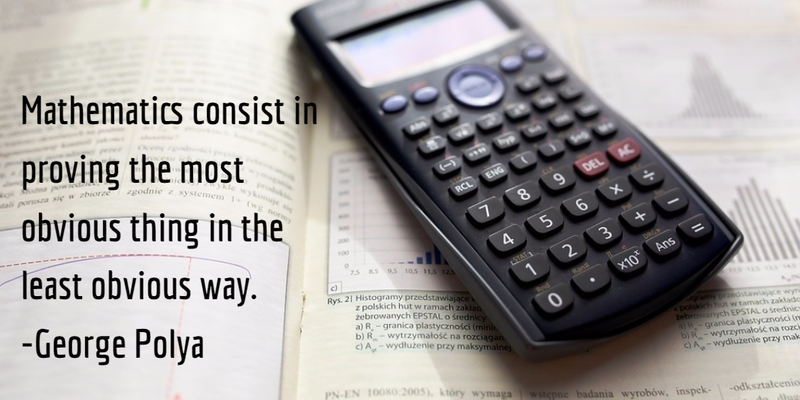23

Is this true?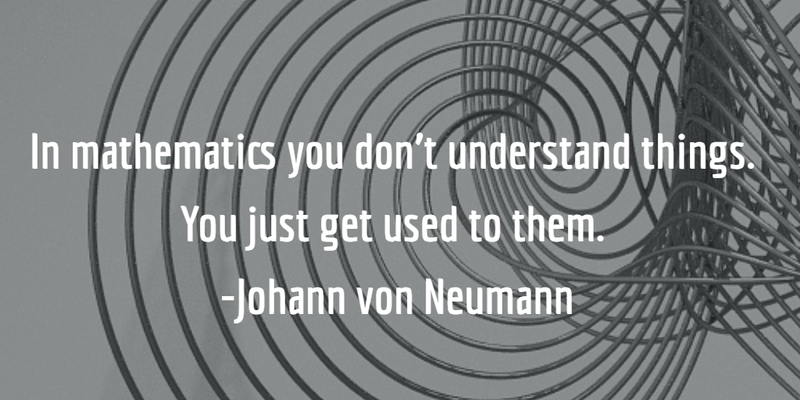24

Simple yet complex.25

Would you agree with these mathematics quotes?